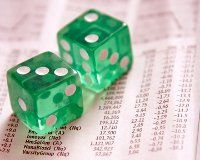# Going long or short - what's the difference?

##Long position

Taking a long CFD position simply means you expect the value of the underlying security to increase. The difference between the entry price and the exit price is the profit or loss that is made on the trade.

For instance, ABC stock is currently trading at \$4 and you expect it to appreciate in value. You have \$10 000 worth of capital and the initial margin required to trade ABC is 5%. You can buy 50 000 ABC shares (with a market value of \$200 000). Two weeks later you sell the shares for \$4.20. They have appreciated 5%.

Your gross profit equals the difference between the entry price and the exit price. In this case it is \$10 000 (20 cents times 50 000 shares). You will need to subtract the financing cost of holding the position two weeks plus the commission required to open and close the CFD. Let?s say the financing cost is 6% p.a. calculated daily and the commission is 0.1%. Therefore your net profit will be approximately \$9 220.32 after you subtract \$410 worth of commission and \$369.68 interest (*).

## Short position

Taking a short CFD position simply means you expect the value of the underlying security to decrease.

For instance, ABC stock is currently trading at \$4 and you expect it decrease in value. You have \$10 000 worth of capital and the initial margin required to trade ABC is 5%. You sell 50 000 ABC shares (with a market value of \$200 000). Two weeks later you buy back the shares for \$3.80. They have depreciated 5%.

Your gross profit is the difference between the entry price where you sold ABC shares and the exit price where you purchased them back. In this case your gross profit is again, \$10 000 (20 cents times 50 000 shares). Because it is a short position you will need to add the interest received on holding the position two weeks and subtract the commission required to open and close the CFD. Again, let’s assume the interest rate is 6% p.a. calculated daily and the commission is 0.1%. Therefore your net profit will be approximately \$9 963.61 after you subtract \$390 worth of commission and add \$353.61 interest.

Long Short
Rate 6% 6%
Commission 0.10% 0.10%
Present Value \$200 000 \$200 000
Open CFD Position Fee \$200 \$200
Future Value \$210 000 \$190 000
Close CFD Position Fee \$210 \$190
Gross Profit \$10,000 \$10,000
minus fees \$410 \$390
minus interest (long) \$369.68